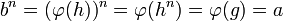# Divisibility is quotient-closed

Suppose$G$ is a group and$H$ is a normal subgroup of$G$, so that$G/H$ is the quotient group. Then, if$n$ is a natural number such that$G$ is$n$-divisible (i.e., every element of$G$ has a$n^{th}$ root in$G$, then so is$G/H$, i.e., every element of$G/H$ has a$n^{th}$ root in$H$.
Given: A group$G$ and a natural number$n$ such that every element of$G$ has a$n^{th}$ root in$G$, a normal subgroup$H$ with quotient group$G/H$. An element$a \in G/H$.
To prove: There exists$b \in G/H$ such that$b^n = a$.
Proof: Let$\varphi:G \to G/H$ be the quotient map and let$g \in G$ be such that$\varphi(g) = a$. Since$G$ is$n$-divisible, there exists$h \in G$ such that$h^n = g$. Let$b = \varphi(h)$. Then,$b^n = (\varphi(h))^n = \varphi(h^n) = \varphi(g) = a$, so$b$ is as desired.